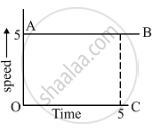# A Body is Moving Uniformly in a Straight Line with a Velocity of 5 M/S. Find Graphically the Distance Covered by It in 5 Seconds. - Science

A body is moving uniformly in a straight line with a velocity of 5 m/s. Find graphically the distance covered by it in 5 seconds.

#### Solution

We have to calculate the distance travelled by the moving body whose speed time graph is given to us.
Distance travelled = Area of rectangle OABC
So, distance travelled,
= (OA)(OC)
= (5)(5) m
= 25 mConcept: Velocity - Time Graphs
Is there an error in this question or solution?

#### APPEARS IN

Lakhmir Singh Science Class 9 Physics
Chapter 1 Motion
Hots Questions | Q 57 | Page 43